# Regression Analysis 202 - Stability Test

This is the fourth entry in our regression analysis and modeling series. In this tutorial, we continue the analysis discussion we started earlier and leverage an advanced technique –regression stability test - to help us detect deficiencies in the selected model, and thus the reliability of the forecast.

Again, we will use a sample data set gathered from 20 different salespersons. The regression model attempts to explain and predict weekly sales for each salesperson (dependent variable) using two explanatory variables: intelligence (IQ) and extroversion.

## Data Preparation

Similar to what we did in an earlier tutorial, we organize our sample data by placing the value of each variable in a separate column and each observation in a separate row.

In this example, we have 20 observations and two independent (explanatory) variables. The response or dependent variable is the weekly sales.

Next, we introduce the “mask”. The “mask” is a Boolean array (0 or 1), which chooses which variable is included (or excluded) from the analysis.

Let’s use the results from the 3rd entry in this tutorial series, and set the mask entry for “Intelligence” to be 0 and the extroversion to be 1.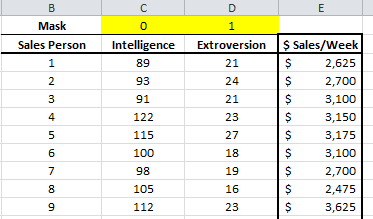Furthermore, let’s exclude observation #16, as it proved to be influential on our regression model.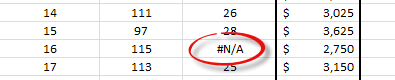## Process

To examine the stability of the regression model, we need to split the data set into two non‐overlapping data sets: data set 1 and data set 2.

The regression stability test constructs 3 different regressions models.

1. Model 1: Using observations in data set 1: $$Y = \alpha_1 + \beta_{1,1}X_{1,i}+\beta_{1,2}X_{2,i} +\cdots + \beta_{1,p}X_{p,i}$$
2. Model 2: Using observations in data set 2: $$Y = \alpha_2 + \beta_{2,1}X_{1,i}+\beta_{2,2}X_{2,i} +\cdots + \beta_{2,p}X_{p,i}$$
3. Model 3: Using observations in data 1 and in data set 2: $$Y = \alpha_3 + \beta_{3,1}X_{1,i}+\beta_{3,2}X_{2,i} +\cdots + \beta_{3,p}X_{p,i}$$

Ask the following question: $$H_o=\left\{\begin{matrix} \alpha_1 =\alpha_2 = \alpha & \\ \beta_{1,j}=\beta_{2,j}=\beta_j & \end{matrix}\right.$$ $$H_1=\left\{\begin{matrix} \exists \alpha_i \neq \alpha ∓ \\ \beta_{i,j} \neq \beta_j & \end{matrix}\right.$$ $$1 \leq i \leq 2$$ $$1 \leq j \leq p$$

In plain English, is any of the regression coefficients value in either data set significantly different from that in the other data set or the combined data set?

For our demonstration purposes, we will choose the first 10 observations as data set 1, and the remaining as data set 2 (11 to 20). Draw a line to outline the separation.

Now we are ready to conduct our regression stability analysis.

• Select an empty cell in your worksheet where you wish the test output to be generated.
• Locate and click on the “Statistical Test” icon, select the regression stability, and click on the “Regression” icon in the NumXL tab (or toolbar).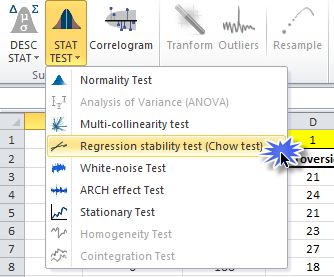• The Regression Stability Test Wizard appears. Select the input cell range for data set 1 and data set 2. Specify the input mask: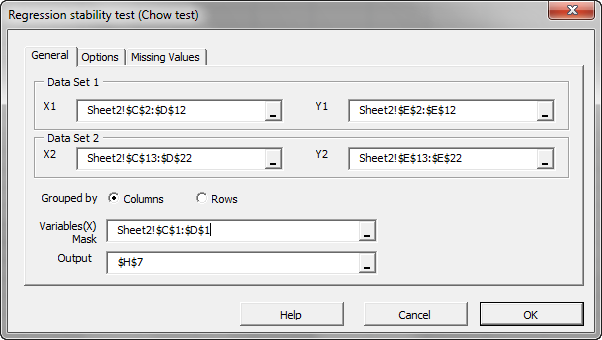• In the “Options” and “Missing Values” tabs, accept the defaults.
• Click “OK.”
• The Chow test table is generated.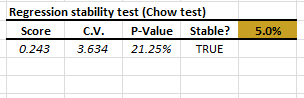The Chow test accepts (or does not reject) the null hypothesis that the values of the coefficients are statistically indifferent in the entire data set.

## Conclusion

In this tutorial, we explored the stability of the regression in the input sample data.

Based on our findings, the model can be used for delivering a forecast using one explanatory variable (i.e. extroversion).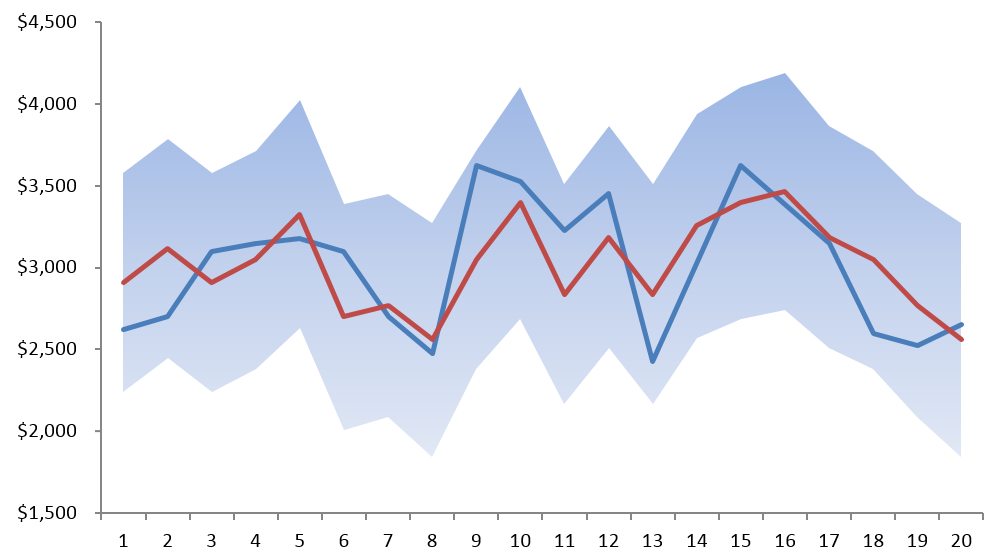Finally, we may wonder whether we can still improve (i.e. reduce the regression error) by combining the two explanatory variables (intelligence and extroversion)?

Answer: Maybe, but this is a topic for a different series – specifically principal component regression (PCR).